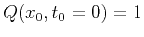Time-to-depth conversion and seismic velocity estimation using time-migration velocityNext: Inversion Methods Up: Partial differential equations for Previous: 3-D case

## Cauchy problem for elliptic equations

Equations 14 and 20 reveal the nature of the instabilities in the problem in hand. These PDEs are elliptic, while the physical setting allows us to pose only a Cauchy problem for them, which is well-known to be ill-posed. Furthermore, the fact that the PDEs involve not only the Dix velocity itself, but also its first and second derivatives, leads to high sensitivity of the solutions to input data.

Nonetheless, we found two ways for solving these PDEs numerically on the required, and relatively short, interval of time: namely, a finite difference scheme inspired by the Lax-Friedrichs method and a spectral Chebyshev method. A detailed analysis of the problem shows that our methods work thanks to

1. the special input, corresponding to a positive finite seismic velocity;
2. the special initial conditions,corresponding to the image rays;
3. the fact that our finite difference method contains error terms which damp the high harmonics; truncation of the polynomial series in the spectral Chebyshev method which is similar to truncation of the high harmonics; and
4. the short interval of time, in which we need to compute the solution, so that the growing low harmonics fail to develop significantly.
Items 1 and 2 say that the exact solutions of our PDEs for the hypothetical perfect Dix velocity given by equations 10 and 11 are finite and nonzero. Items 3 and 4 say that the numerical methods take care of the imperfection of the data and computations on a short enough time interval.Time-to-depth conversion and seismic velocity estimation using time-migration velocityNext: Inversion Methods Up: Partial differential equations for Previous: 3-D case

2013-03-02